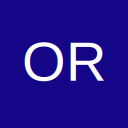# Shape paint with macro

• Hi guys,

I have macro code to paint several shapes different colors accordingly the conditions. With one of the condition need to make pattern change as well but i couldn't do it. Could you please help about it to include any of them how to add patter change as well.

thank you so much

Sub Status()

On Error Resume Next

For c = 1 To 5000

'Empty
If Worksheets("Status").Cells(c, 1).Value = "R90" And Worksheets("Status").Cells(c, 9).Value = "" Then Worksheets("R90").Shapes(Cells(c, 3).Value).Fill.ForeColor.RGB = RGB(255, 255, 255)

'WORK FRONT
If Worksheets("Status").Cells(c, 1).Value = "R90" And Worksheets("Status").Cells(c, 9).Value = "WORK FRONT" Then Worksheets("R90").Shapes(Cells(c, 3).Value).Fill.ForeColor.RGB = RGB(0, 176, 240)

'IN PROCESS
If Worksheets("Status").Cells(c, 1).Value = "R90" And Worksheets("Status").Cells(c, 9).Value = "IN PROCESS" Then Worksheets("R90").Shapes(Cells(c, 3).Value).Fill.ForeColor.RGB = RGB(0, 176, 80)

'FINISHED
If Worksheets("Status").Cells(c, 1).Value = "R90" And Worksheets("Status").Cells(c, 9).Value = "FINISHED" Then Worksheets("R90").Shapes(Cells(c, 3).Value).Fill.ForeColor.RGB = RGB(146, 208, 80)

Next c

End Sub

## Participate now!

Don’t have an account yet? Register yourself now and be a part of our community!